> 文档中心 > 为什么C++中的继承比Java设计的要复杂，原因竟出在这儿？

# 为什么C++中的继承比Java设计的要复杂，原因竟出在这儿？你好，我是安然无虞。

### 文章目录

• 继承的概念及定义
• 继承的概念
• 继承的定义
• 定义格式
• 继承关系和访问限定符
• 继承基类成员访问方式的变化
• 基类和派生类对象赋值转换
• 继承中的作用域
• 派生类的默认成员函数
• 继承与友元
• 继承与静态成员
• 复杂的菱形继承和菱形虚拟继承
• 虚拟继承解决数据冗余和二义性的原理
• 继承的总结和反思
• 继承和组合
• 笔试面试题

# 继承的概念及定义

## 继承的概念

``class Student{string _name;//姓名string _tel;//电话string _address;//地址int _age;//年龄//……string _stuID;//学号};``

``class Teacher{string _name;string _tel;string _address;int _age;//……string _workID;//工号};``

``class Person{string _name;string _tel;string _address;int _age;};``

Student类：

``class Studet : public Person{string _stuID;};``

Teacher类：

``class Teacher : public Person{string _workID;};``

``class Person{public:void Print(){cout << "name:" << _name << endl;cout << "age:" << _age << endl;cout << endl;}protected:string _name = "SL";//这是C++11的新玩法int _age = 18;//不是初始化，给的是缺省值};//继承后父类的Person成员的成员变量和成员函数都会变成子类的一部分//这里体现出了Student类和Teacher复用了Person类的成员class Student : public Person{protected:int _stuID;};class Teacher : public Person{protected:int _workID;};int main(){Student s;Teacher t;s.Print();t.Print();return 0;}``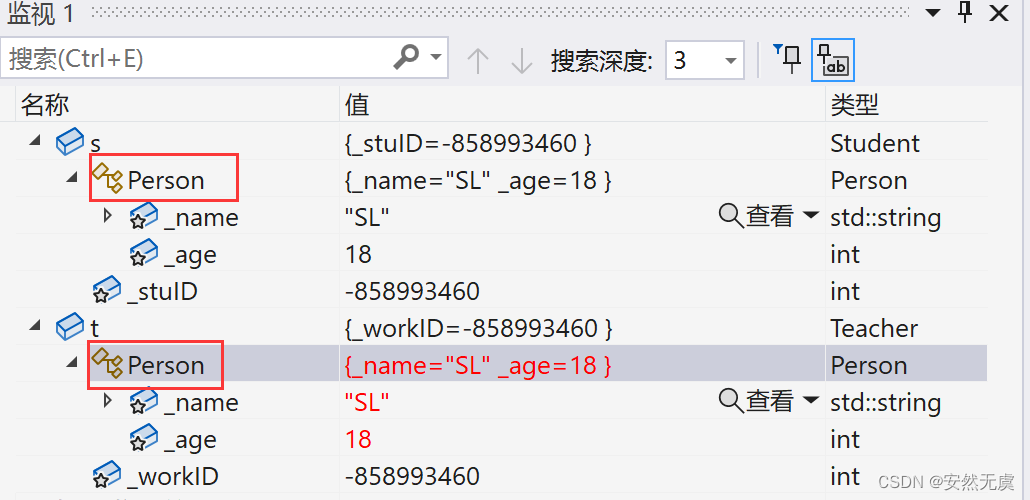## 继承的定义

### 定义格式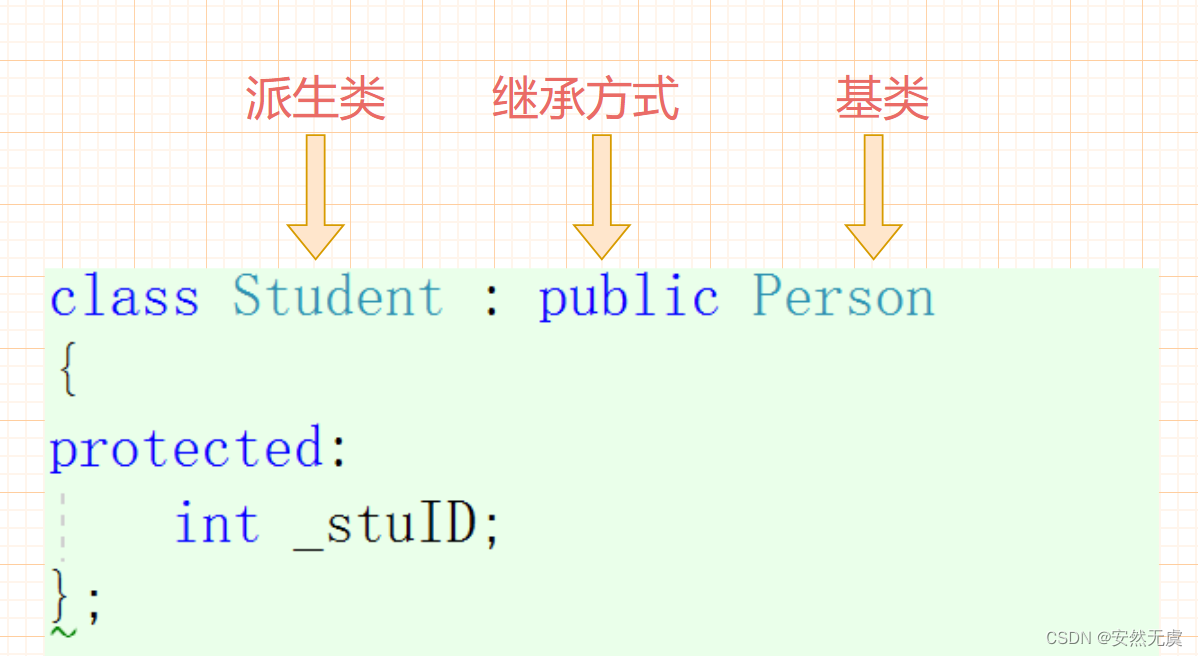• public继承
• protected继承
• private继承

• public访问
• protected访问
• private访问

### 继承基类成员访问方式的变化

1. 基类private成员在派生类中无论以什么继承方式都是不可见的，这里不可见指的是语法上限制了派生类对象不管在类里面还是在类外面都不能访问它，但是基类的私有成员还是继承到了派生类当中
2. 基类的private成员在派生类中是不能被访问的，如果基类成员不想在类外直接被访问，但是需要在派生类当中能被访问，就需要定义为protected成员。这个时候就能发现，原来protecte访问限定符是为了继承而设置的；
3. 对上面的表格进一步总结就会发现，基类的私有成员在子类当中都是不可见的，基类的其他成员在子类当中的访问方式 == 成员在基类当中的访问限定符和继承方式，二者取小的那一个，注意哦，这个是继承原则；
4. 使用关键字class时默认继承方式是private，使用struct时默认继承方式是public，不过最好显示的写出继承方式；
5. 在实际运用当中一般都是public继承，几乎很少使用protected/private继承，现实中也不提倡使用，因为protected/private继承下来的成员都只能在派生类里面使用，实际中的扩展维护性不强，大佬早期设计的时候想复杂了，实际当中的基类成员都是保护或者公有，继承方式都是公有继承

# 基类和派生类对象赋值转换

1.派生类对象可以赋值给基类的对象/基类的指针/基类的引用。这里有个很形象的说法叫切片或切割，寓意是把派生类中基类那部分切来赋值过去。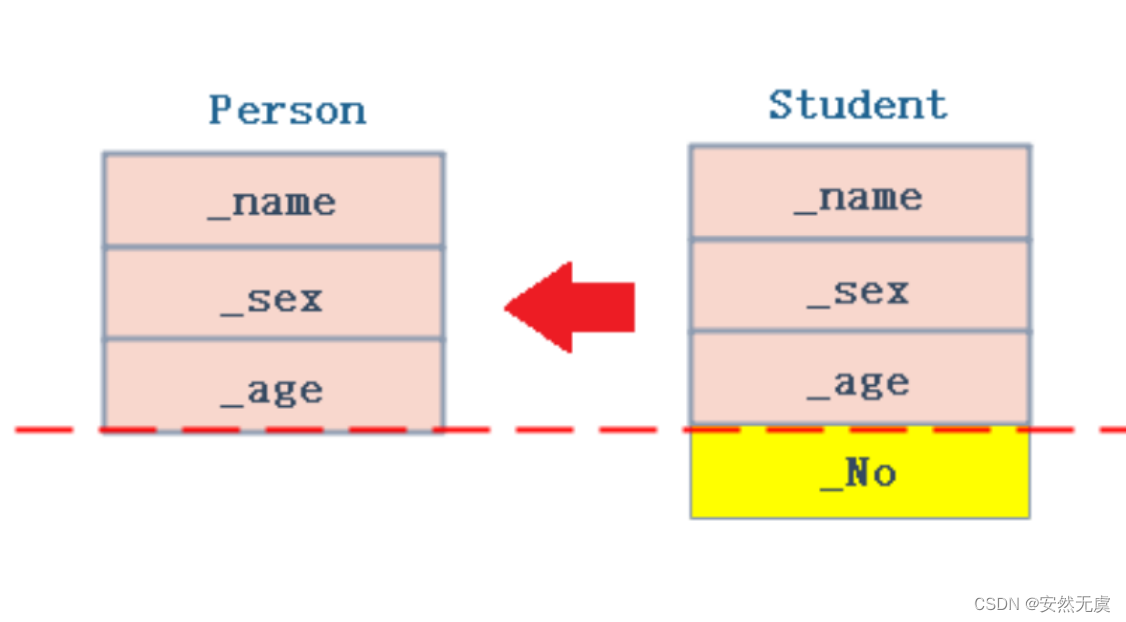2.基类对象不能赋值给派生类对象

3.基类对象的指针或引用可以通过强制类型转换赋值给派生类对象的指针或引用，但是必须是基类对象的指针必须指向派生类对象时才是安全的。（具体放到后面讲解，这里大家知道有这点即可）

``class Person{protected:string _name;string _sex;int _age;};class Student : public Person{public:int _No;//学号};void Test(){Student s;//1、派生类对象可以赋值给基类对象/指针/引用Person p = s;Person* pp = &s;Person& rp = s;//2、基类对象不能赋值给派生类对象，注意哦，强制类型转换也不可以//s = p;//3、基类指针可以通过强制类型转换赋值给派生类指针，但有些情况会导致越界，后面会详细说明pp = &s;Student* ps1 = (Student*)pp;}``

# 继承中的作用域

1.在继承体系中基类和派生类都有独立的作用域

2.基类和派生类中有同名成员，派生类成员将屏蔽基类对同名成员的直接访问，这种情况叫隐藏，也叫重定义（在派生类成员函数中，可以使用 基类::基类成员 显示访问）；

``//Student的_num和Person的_num构成隐藏关系，很容易混淆class Person{protected:string _name = "张三";int _num = 168;//身份证号};class Student : public Person{public :void Print(){cout << "姓名：" << _name << endl;cout << "身份证号：" << Person::_num << endl;//指定作用域cout << "学号：" << _num << endl;}protected:int _num = 999;//学号};``

3.需要注意如果是成员函数的隐藏，只需要函数名相同就构成隐藏；

4.注意实际当中的继承体系里不要定义同名的成员（包括成员变量和成员函数）。

``//B中的fun()和A中的fun()不是构成重载，因为不是在同一作用域//基类和派生类都有各自独立的作用域//B中fun()和A中fun()构成隐藏，成员函数满足函数名相同构成隐藏关系class A{public :void fun(){cout << "fun()" << endl;}};class B : public A{public :void fun(int i){A::fun();cout << "fun(int i)" << endl;}};void Test(){B b;b.fun(10);}``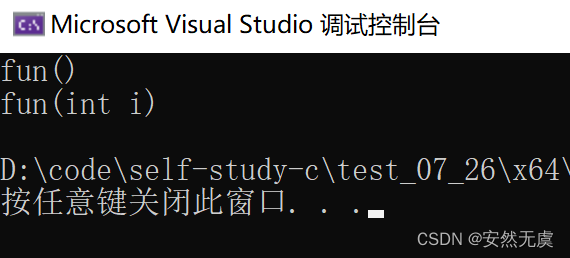``void Test(){B b;b.fun();//编译报错，A中的fun()函数被隐藏了，默认调用的是派生类的成员函数，改成b.A::fun()才正确b.fun(10);}``

# 派生类的默认成员函数

6个默认成员函数，“默认”的意思就是我们不写，编译器也会自动生成一个，那么在派生类中，这几个成员函数是如何生成的呢？

1、派生类的构造函数必须调用基类的构造函数初始化基类的那一部分成员。如果基类没有默认的构造函数，则必须在派生类构造函数的初始化列表阶段显示调用；

2、派生类的拷贝构造函数必须调用基类的拷贝构造函数完成基类的拷贝初始化；

3、派生类的operator=必须要调用基类的operator=完成基类的复制；

4、派生类的析构函数会在被调用完成后自动调用基类的析构函数清理基类成员。因为这样才能保证派生类对象先清理派生类成员再清理基类成员的顺序。

5、派生类对象初始化先调用基类构造再调用派生类构造；

6、派生类对象析构清理先调用派生类析构再调用基类析构；

7、因为后续一些场景析构函数需要构成重写，重写的条件之一是函数名相同，那么编译器会对析构函数名进行特殊处理，处理成destrutor()，所以基类析构函数在不加virtual的情况下，派生类析构函数和基类析构函数构成隐藏关系。（这点在后面多态部分详细讲解）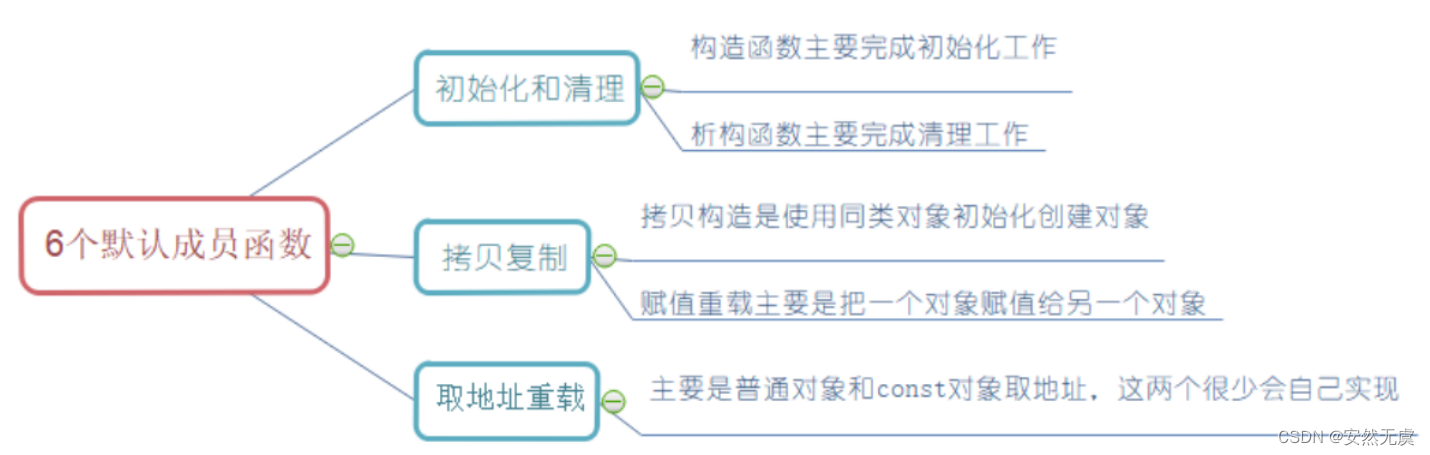``class Person{public:Person(const char* name = "张三"):_name(name){cout << "Person()" << endl;}Person(const Person& p):_name(p._name){cout << "Person(const Person& p)" << endl;}Person& operator=(const Person& p){cout << "Person operator=(const Person& p)" << endl;if (this != &p)_name = p._name;return *this;}~Person(){cout << "~Person()" << endl;}protected:string _name;};class Student : public Person{public://初始化列表初始化顺序是按照声明的顺序，一般我们认为父类是在前声明的//所以即使改成:_num(num) ,Person(name)也是先初始化父类的对象Student(const char* name = "", int num = 0):Person(name)//注意这里不是_name(name)，父类一定要调用父类的默认构造初始化,_num(num){cout << "Student()" << endl;}//子类构造函数原则:（析构、拷贝、赋值也类似）//a.调用父类构造函数初始化继承自父类成员；//b.自己再初始化自己成员Student(const Student& s):Person(s)//调用父类的默认构造初始化父类的那一部分,_num(s._num){cout << "Student(const Student& s)" << endl;}Student& operator=(const Student& s){cout << "Student& operator=(const Student& s)" << endl;if (this != &s){Person::operator=(s);_num = s._num;}return *this;}~Student(){//~Person();//报错——父子类析构函数构成隐藏关系（多态部分讲解原因）//试想：显示调用Person::~Person()，这样也是错误的，原因是没必要//为了保证析构顺序，先子后父，子类析构函数完成后会自动调用父类的析构函数，所以不需要我们显示调用cout << "~Student()" << endl;}protected:int _num;//学号};void Test(){Student s1("张三", 20510);Student s2(s1);s1 = s2;}``

``class A{private:A(){}};//父类的构造函数私有化后，子类就无法构造对象class B : public A{}``

# 继承与友元

``class Student;class Person{public:friend void Display(const Person& p, const Student& s);protected:string _name;};class Student : public Person{protected:int _stuNum;//学号};void Display(const Person& p, const Student& s){cout << p._name << endl;cout << s._stuNum << endl;//报错}``

# 继承与静态成员

``class Person{public:Person(){++_count;}protected:string _name;public:static int _count;//统计人的个数};int Person::_count = 0;class Student : public Person{protected:int _stuNum;//学号};class Graduate : public Student{protected:string _course;//学科};void Test(){Student s1;Student s2;Student s3;Graduate s4;cout << "人数：" << Person::_count << endl;Student::_count = 0;cout << "人数：" << s4._count << endl;cout << "s1._count地址：" << &s1._count << endl;cout << "s4._count地址：" << &s4._count << endl;}``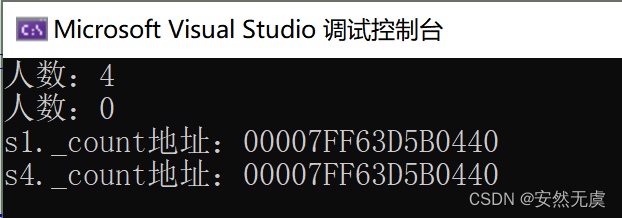# 复杂的菱形继承和菱形虚拟继承

1、单继承：一个子类只有一个直接父类时，称这个继承关系为单继承。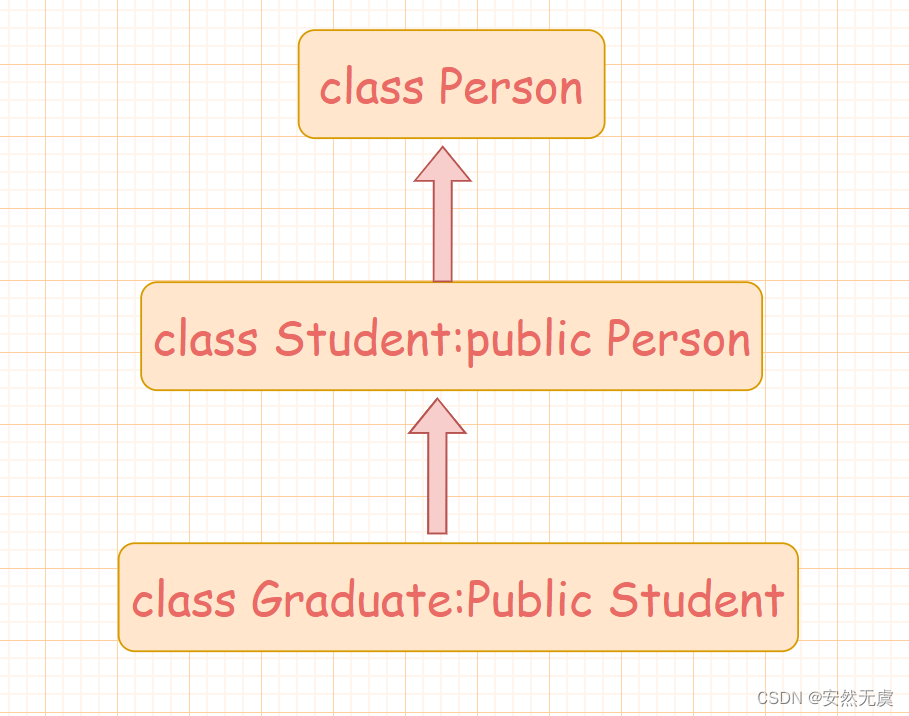2、多继承：一个子类有两个或两个以上的直接父类时，称这个继承关系为多继承。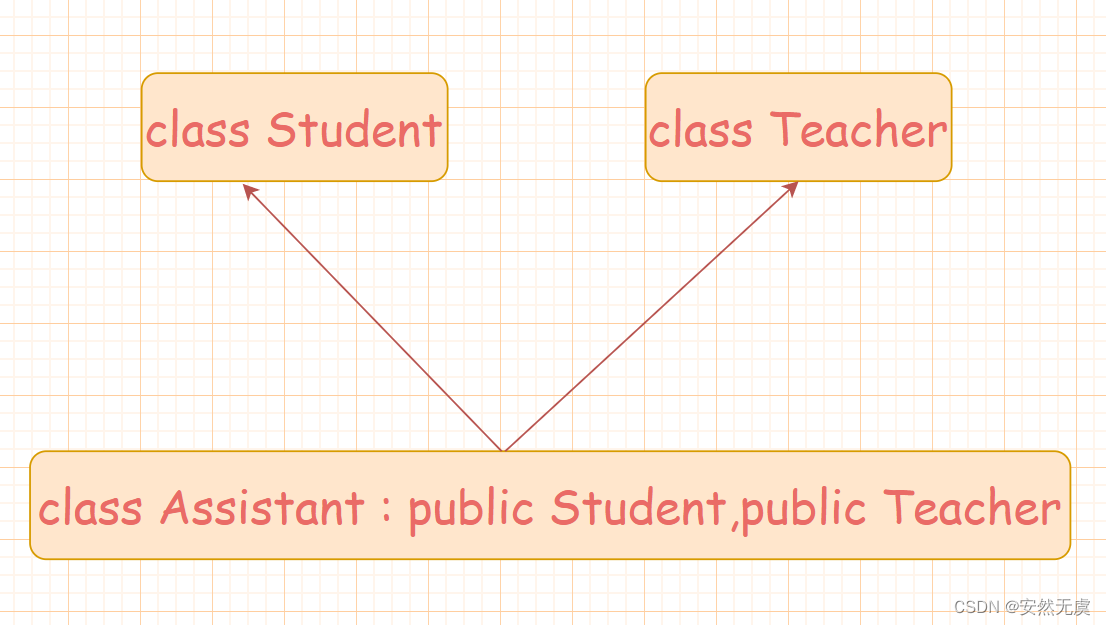3、菱形继承：菱形继承是多继承的一种特殊情况。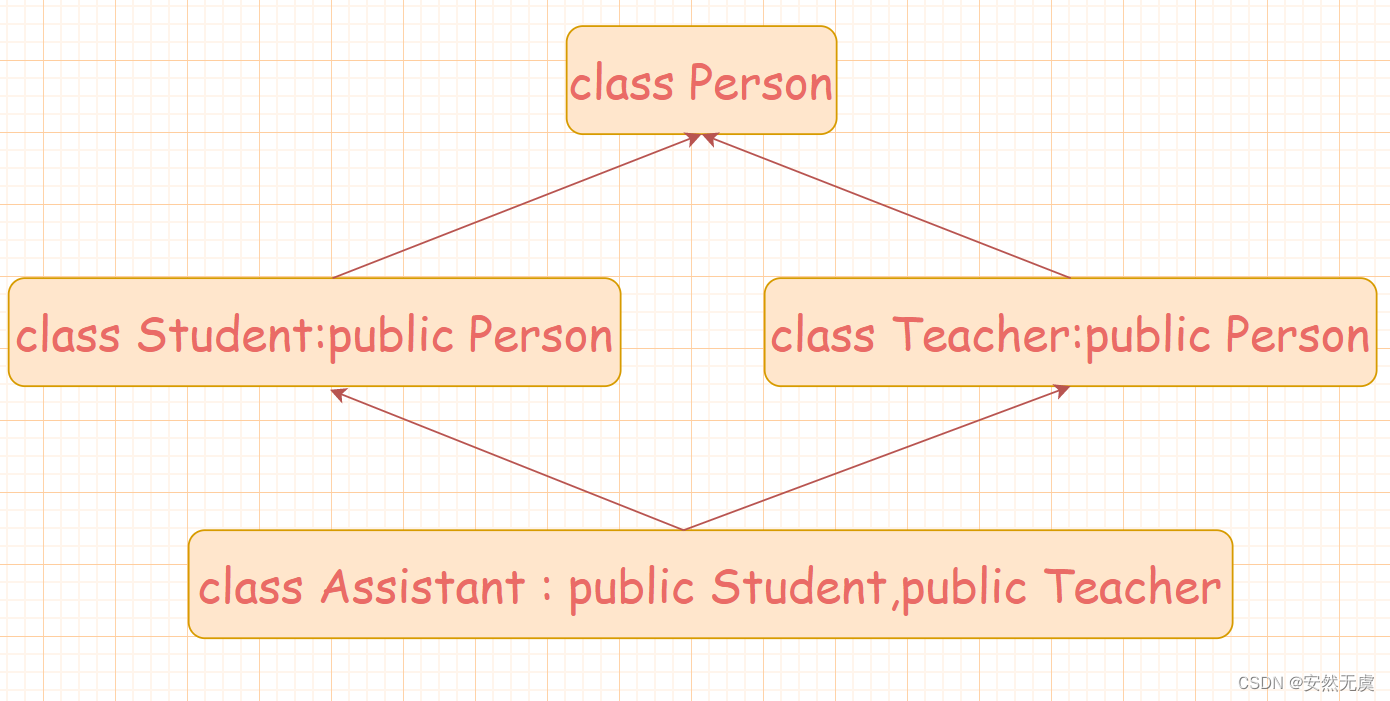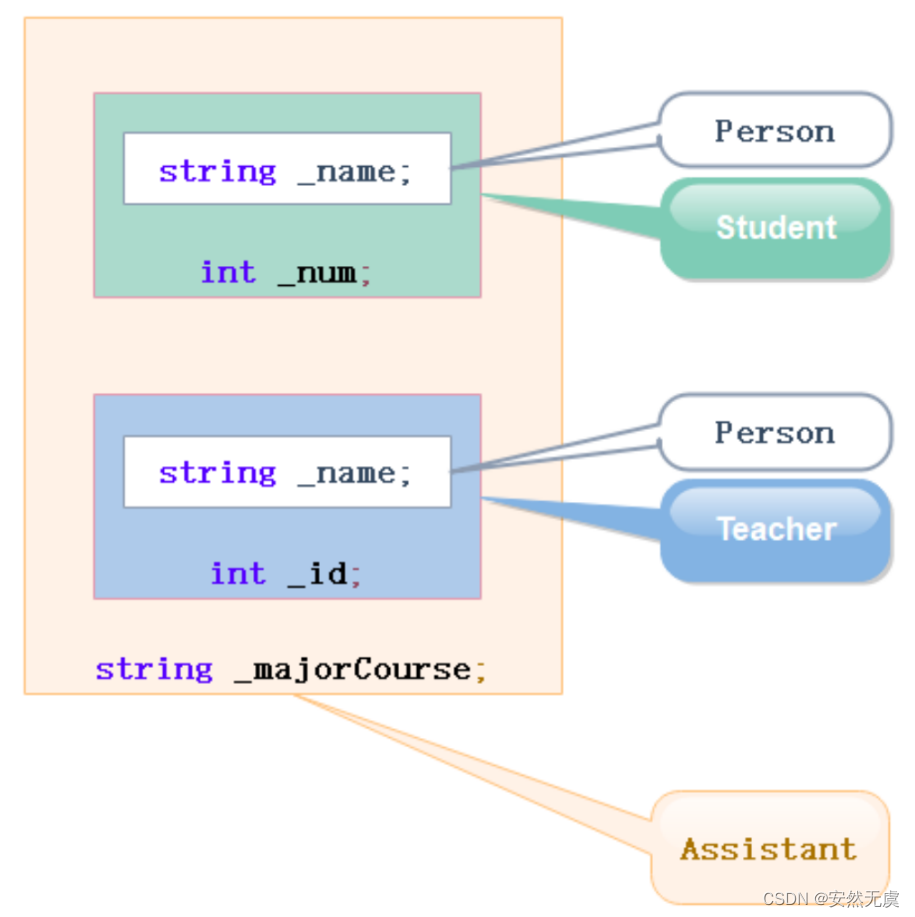``class Person{public:string _name;};class Student : public Person{protected:int _num;//学号};class Teacher : public Person{protected:int _id;//职工编号};class Assistant : public Student, public Teacher{protected:string _majorCourse;//主修课程};void Test(){//存在二义性，不知道访问哪一个Assistant a;//a._name = "诸葛大力";//需要显示指定访问哪个父类的成员可以解决二义性问题，但是数据冗余问题依旧不能解决a.Student::_name = "张三";a.Teacher::_name = "李四";}``

``class Person{public:string _name;};class Student : virtual public Person{protected:int _num;//学号};class Teacher : virtual public Person{protected:int _id;//职工编号};class Assistant : public Student, public Teacher{protected:string _majorCourse;//主修课程};void Test(){Assistant a;a._name = "诸葛大力";}``

## 虚拟继承解决数据冗余和二义性的原理

``class A{public :int _a;};class B : public A{public:int _b;};class C :public A{public:int _c;};class D : public B, public C{public:int _d;};int main(){D d;d.B::_a = 1;d.C::_a = 2;d._b = 3;d._c = 4;d._d = 5;return 0;}``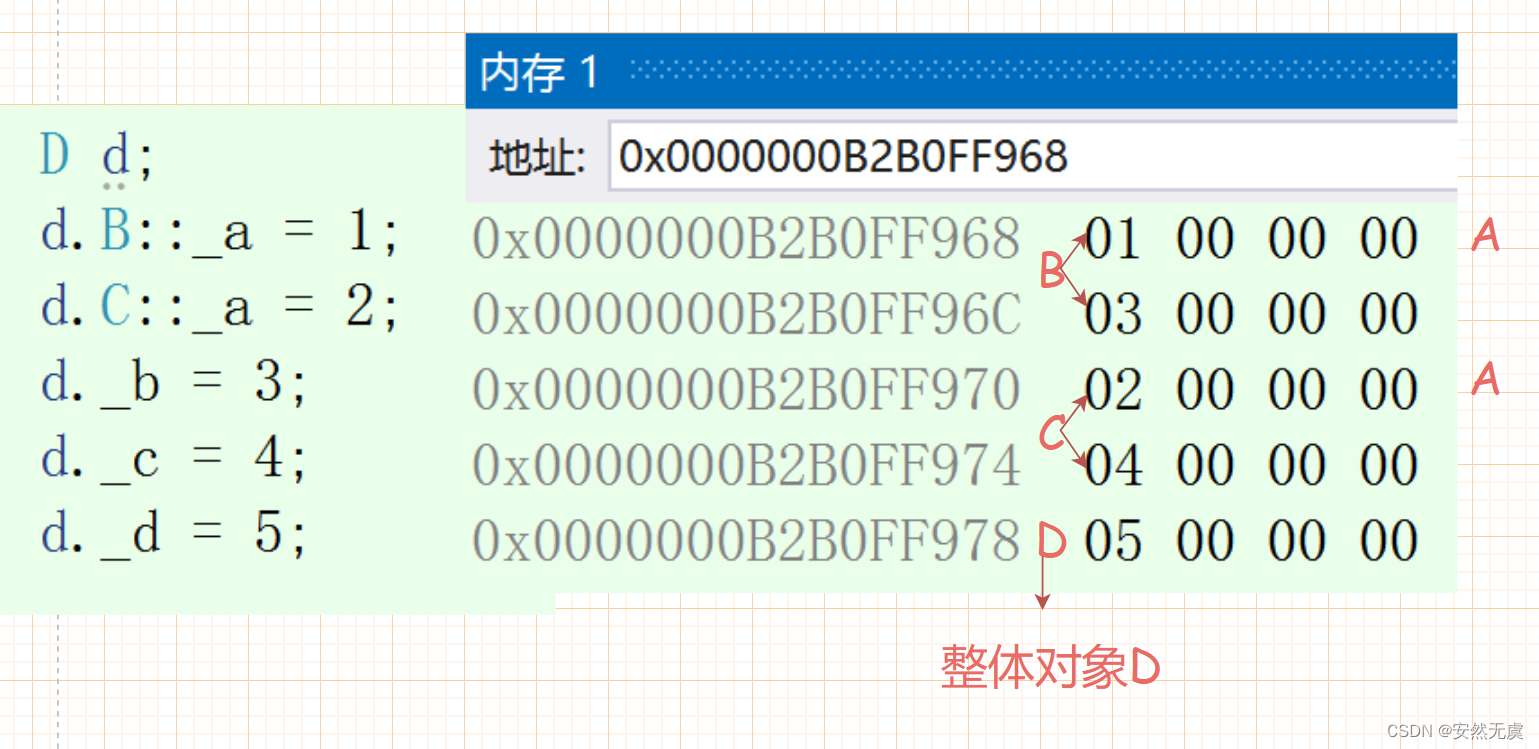``class A{public :int _a;};class B : virtual public A{public:int _b;};class C : virtual public A{public:int _c;};class D : public B, public C{public:int _d;};int main(){D d;d.B::_a = 1;d.C::_a = 2;d._b = 3;d._c = 4;d._d = 5;return 0;}``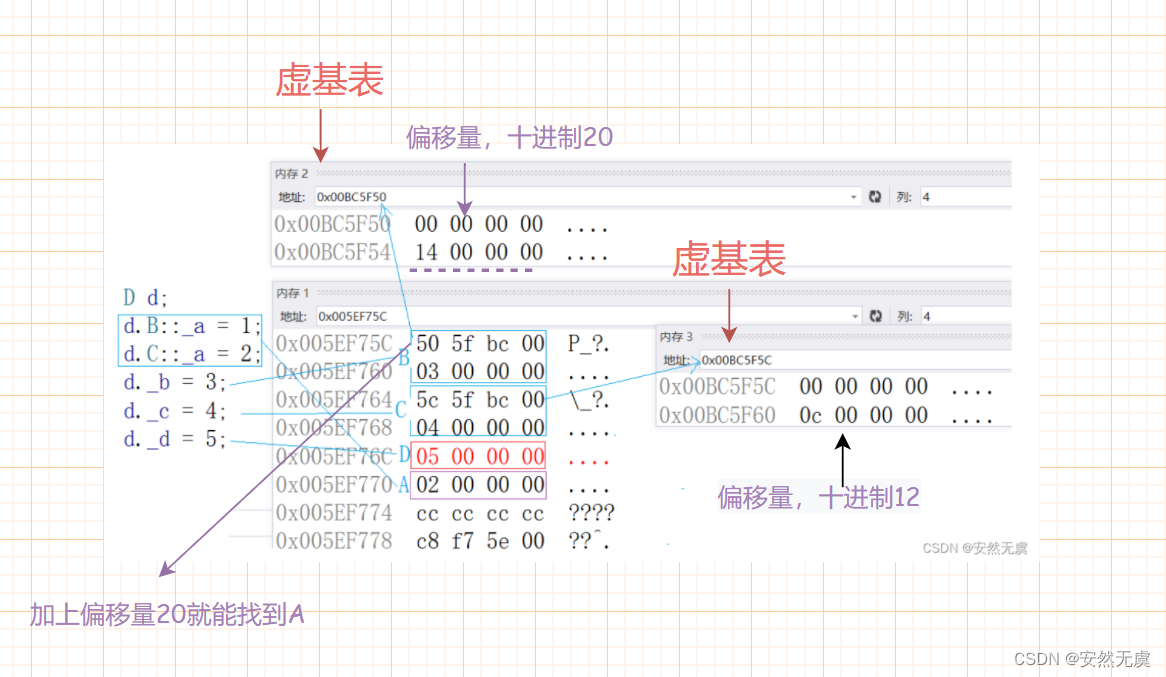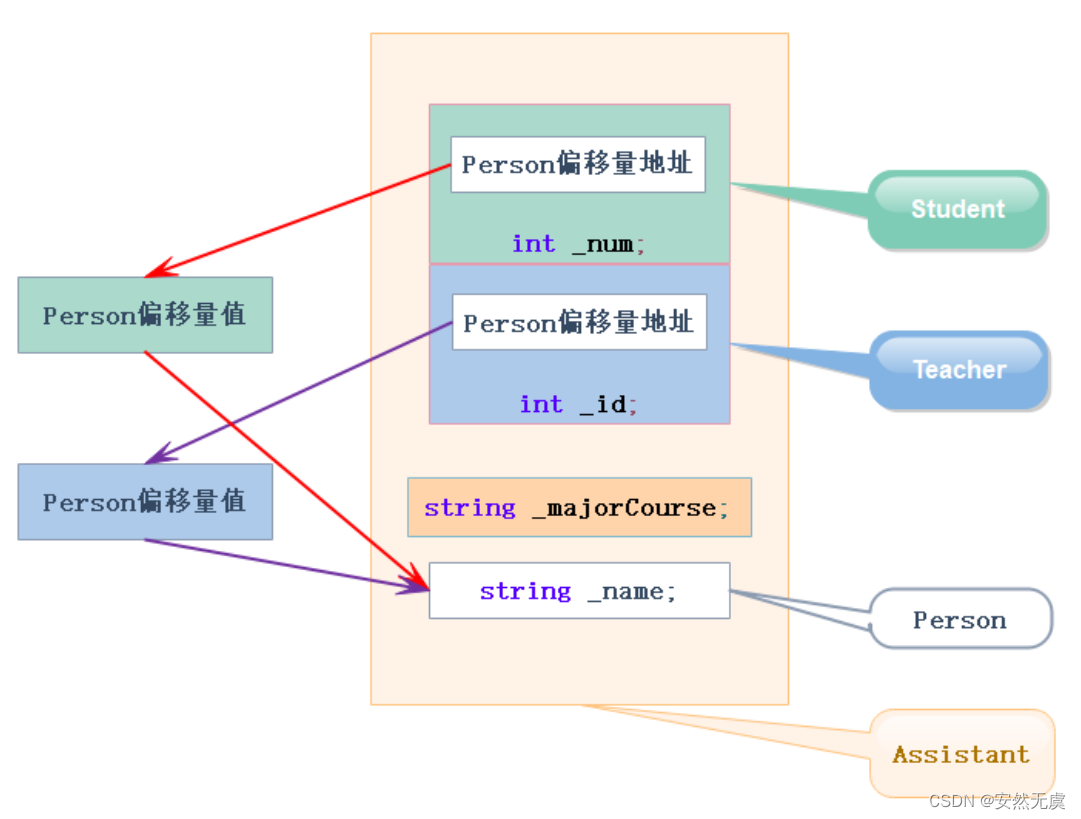``D d;//会切片，通过偏移量来找各自的AB b = d;C c = d;``

# 继承的总结和反思

1、我们都说C++语法复杂，其实多继承就是一个体现。有了多继承，就会存在菱形继承，有了菱形继承就会有菱形虚拟继承，它们的底层实现相当复杂。所以一般不建议使用多继承，更不要使用菱形继承，因为在复杂度和性能上都会存在问题。
2、多继承可以认为是C++的设计缺陷之一，后来很多OOP都没有多继承，比如Java。

## 继承和组合

public 继承是一种 is-a 的关系，也就是说每一个派生类对象都是一个基类对象。比如：学生->人，狗->动物

``class A{};//继承class B : public A{};``

``class C{};//组合class D{C _c;};``

# 笔试面试题

• 什么是菱形继承？菱形继承的问题是什么？
• 什么是菱形虚拟继承？如何解决数据冗余和二义性的？
• 继承和组合的区别？什么时候用继承？什么时候用组合？

​​